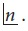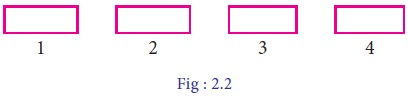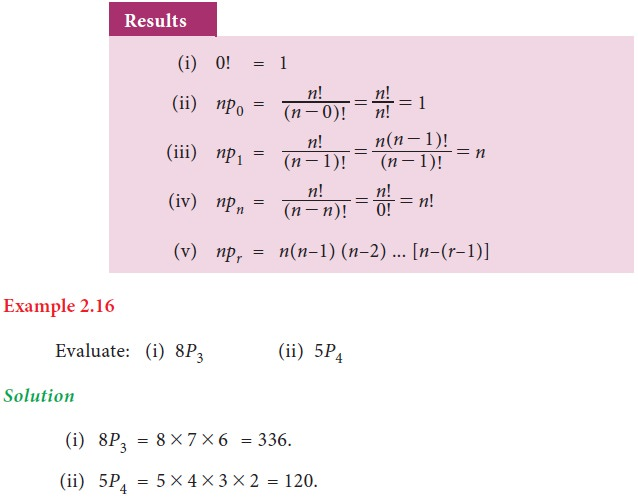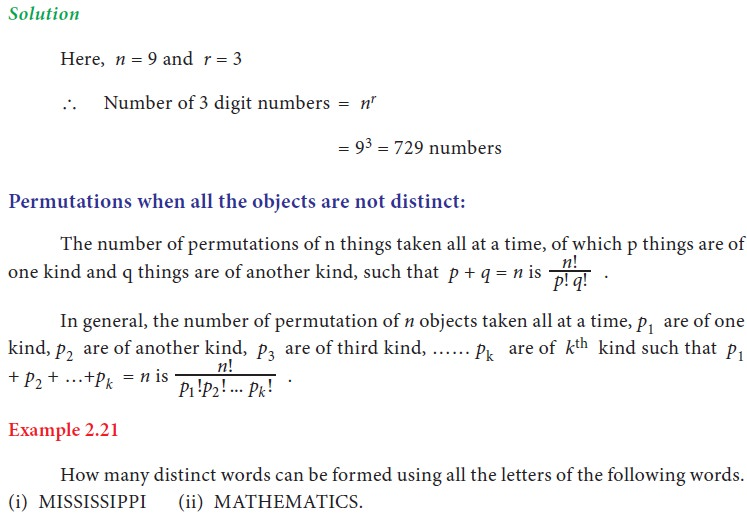Home | | Business Maths 11th std | Permutations

# Permutations

There are two jobs. Of which one job can be completed in m ways, when it has completed in any one of these m ways, second job can be completed in n ways, then the two jobs in succession can be completed in m # n ways. This is called multiplication principle of counting.

Permutations

## 1. Factorial

For any natural number n, n factorial is the product of the first n natural numbers and is denoted by n! or## 2. Fundamental principle of counting

### Multiplication principle of counting:

Consider the following situation in an auditorium which has three entrance doors and two exit doors. Our objective is to find the number of ways a person can enter the hall and then come out.

Assume that, P1, P2 and P3 are the three entrance doors and S1 and S2 are the two exit doors. A person can enter the hall through any one of the doors P1, P2 or P3 in 3 ways. After entering the hall, the person can come out through any of the two exit doors S1 or S 2 in 2 ways.

Hence the total number of ways of entering the hall and coming out is

3 x 2 = 6 ways.

These possibilities are explained in the given flow chart (Fig. 2.1).The above problem can be solved by applying multiplication principle of counting.

Example 2.11

Find the number of 4 letter words, with or without meaning, which can be formed out of the letters of the word “ NOTE”, where the repetition of the letters is not allowed.

Solution

Let us allot four vacant places (boxes) to represent the four letters. N,O,T,E of the given word NOTE.The first place can be filled by any one of the 4 letters in 4 ways. Since repetition is not allowed, the number of ways of filling the second vacant place by any of the remaining 3 letters in 3 ways. In the similar way the third box can be filled in 2 ways and that of last box can be filled in 1 way. Hence by multiplication principle of counting, total number of words formed is 4 x 3 x 2 x 1 = 24 words.

Example 2.12

If each objective type questions having 4 choices, then find the total number of ways of answering the 4 questions.

Solution

Since each question can be answered in 4 ways, the total number of ways of answering 4 questions = 4 x 4 x 4 x 4 =256 ways.

Example 2.13

How many 3 digits numbers can be formed if the repetition of digits is not allowed?

Solution

Let us allot 3 boxes to represent the digits of the 3 digit numberThree digit number never begins with “0”. ` 100’s place can be filled with any one of the digits from 1 to 9 in 9 ways.

Since repetition is not allowed, 10’s place can be filled by the remaining 9 numbers (including 0) in 9 ways and the unit place can be filled by the remaining 8 numbers in 8 ways. Then by multiplication principle of counting, number of 3 digit numbers = 9x9x8 = 648.

## 3. Addition principle of counting

Let us consider the following situation in a class, there are 10 boys and 8 girls. The class teacher wants to select either a boy or a girl to represent the class in a function. Our objective is to find the number of ways that the teacher can select the student. Here the teacher has to perform either of the following two jobs.

One student can be selected in 10 ways among 10 boys.

One student can be selected in 8 ways among 8 girls

Hence the jobs can be performed in 10 + 8 = 18 ways

This problem can be solved by applying Addition principle of counting.

Definition 2.2

If there are two jobs, each of which can be performed independently in m and n ways respectively, then either of the two jobs can be performed in (m+n) ways. This is called addition principle of counting.

Example 2.14

There are 6 books on commerce and 5 books on accountancy in a book shop. In how many ways can a student purchase either a book on commerce or a book on accountancy?

Solution

Out of 6 commerce books, a book can be purchased in 6 ways.

Out of 5 accountancy books, a book can be purchased in 5 ways.

Then by addition principle counting the total number of ways of purchasing any one book is 5+6=11 ways.## 4. Permutation

Suppose we have a fruit salad with combination of “APPLES, GRAPES & BANANAS”. We don’t care what order the fruits are in. They could also be “bananas, grapes and apples” or “grapes, apples and bananas”. Here the order of mixing is not important. Any how, we will have the same fruit salad.

But, consider a number lock with the number code 395. If we make more than three attempts wrongly, then it locked permanently. In that situation we do care about the order 395. The lock will not work if we input 3-5-9 or 9-5-3. The number lock will work if it is exactly 3-9-5. We have so many such situations in our practical life. So we should know the order of arrangement, called Permutation.

Definition 2.3

The number of arrangements that can be made out of n things taking r at a time is called the number of permutation of n things taking r at a time.

For example, the number of three digit numbers formed using the digits 1, 2, 3 taking all at a time is 6

6 three digit numbers are 123,132,231,213,312,321Example 2.17

In how many ways 7 pictures can be hung from 5 picture nails on a wall ?

SolutionExample 2.18

Find how many four letter words can be formed from the letters of the word “LOGARITHMS” ( words are with or without meanings)### Permutation of repeated things:

The number of permutation of n different things taken r at a time, when repetition is allowed is nr.

Example 2.20

Using 9 digits from 1,2,3,……9, taking 3 digits at a time, how many 3 digits numbers can be formed when repetition is allowed?## 5. Circular permutation

In the last section, we have studied permutation of n different things taken all together is n!. Each permutation is a different arrangement of n things in a row or on a straight line. These are called Linear permutation. Now we consider the permutation of n things along a circle, called circular permutation.

Consider the four letters A, B, C, D and the number of row arrangement of these 4 letters can be done in 4! Ways. Of those 4! arrangements, the arrangements ABCD, BCDA, CDAB, DABC are one and the same when they are arranged along a circle.Tags : Introduction, Definition, Formula, Solved Example Problems, Exercise | Mathematics , 11th Business Mathematics and Statistics(EMS) : Chapter 2 : Algebra
Study Material, Lecturing Notes, Assignment, Reference, Wiki description explanation, brief detail
11th Business Mathematics and Statistics(EMS) : Chapter 2 : Algebra : Permutations | Introduction, Definition, Formula, Solved Example Problems, Exercise | Mathematics